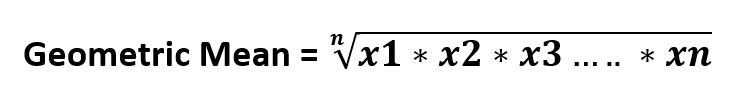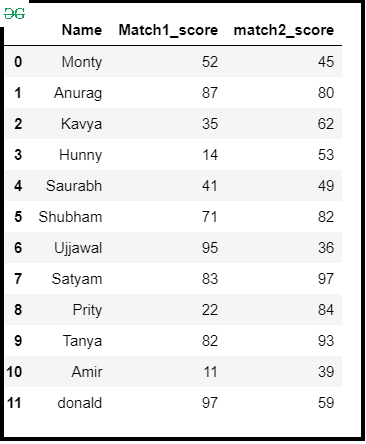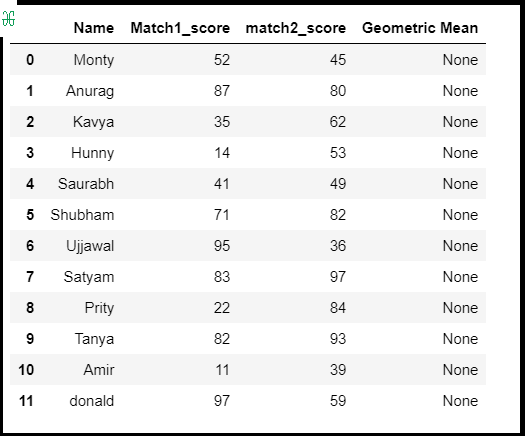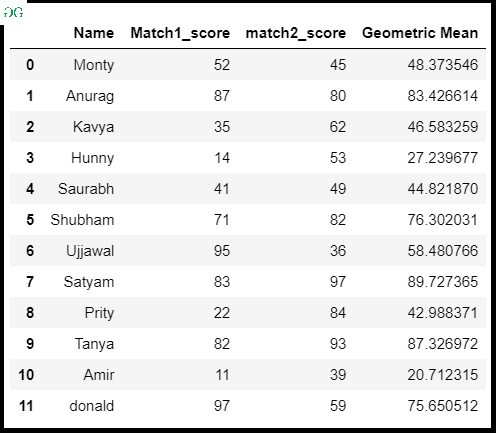# Find the geometric mean of a given Pandas DataFrame

• Last Updated : 05 Sep, 2020

In this article, we will discuss how to find the geometric mean of a given DataFrame. Generally geometric mean of nth numbers is the nth root of their product.Attention geek! Strengthen your foundations with the Python Programming Foundation Course and learn the basics.

To begin with, your interview preparations Enhance your Data Structures concepts with the Python DS Course. And to begin with your Machine Learning Journey, join the Machine Learning - Basic Level Course

It can found using the scipy.stats.gmean() method. This function calculates the geometric mean of the array elements along the specified axis of the array (list in python).

Syntax:

`scipy.stats.gmean(array, axis=0, dtype=None)`

Approach :

• Import module
• Create Pandas DataFrame
• Create a new column for the geometric mean
• Find the geometric mean with scipy.stats.gmean()
• Store into a new column
• Display DataFrame

Step-by-Step Implementation :

Step 1: Importing module and Making Dataframe.

## Python

 `# importing module``import` `pandas as pd``import` `numpy as np``from` `scipy ``import` `stats`` ` `# Create a DataFrame``df ``=` `pd.DataFrame({``    ``'Name'``: [``'Monty'``, ``'Anurag'``, ``'Kavya'``, ``'Hunny'``, ``'Saurabh'``,``             ``'Shubham'``, ``'Ujjawal'``, ``'Satyam'``, ``'Prity'``, ``'Tanya'``, ``             ``'Amir'``, ``'donald'``],``    ``'Match1_score'``: [``52``, ``87``, ``35``, ``14``, ``41``, ``71``, ``95``, ``83``, ``22``, ``82``, ``11``, ``97``],``    ``'match2_score'``: [``45``, ``80``, ``62``, ``53``, ``49``, ``82``, ``36``, ``97``, ``84``, ``93``, ``39``, ``59``]})`` ` `# Display DataFrame``df`

Output :Step 2:  Create an empty DataFrame column.

## Python3

 `# Creating empty column in DataFrame``df[``'Geometric Mean'``] ``=` `None``df`

Output :Step 3: Find Geometric mean with scipy.stats.gmean()  and store it into a new column.

## Python3

 `# Computing geometric mean``# Storing into a DataFrame column``df[``'Geometric Mean'``] ``=` `stats.gmean(df.iloc[:, ``1``:``3``], axis``=``1``)``df`

Output :My Personal Notes arrow_drop_up